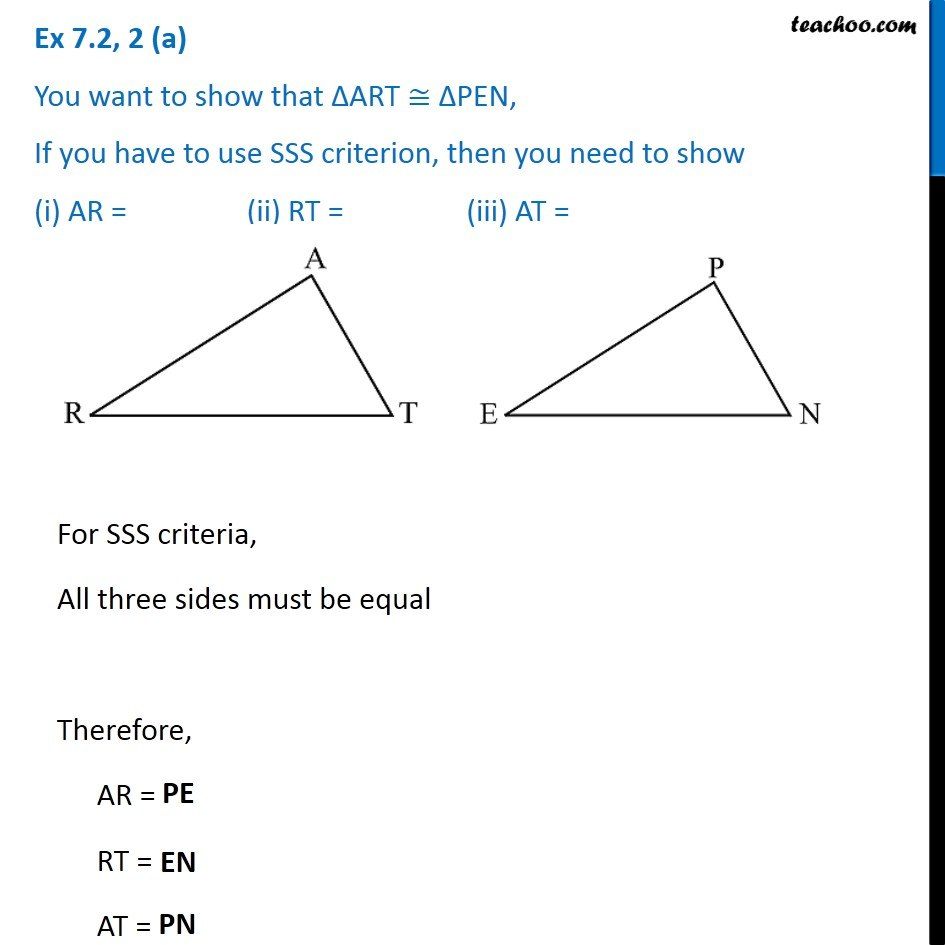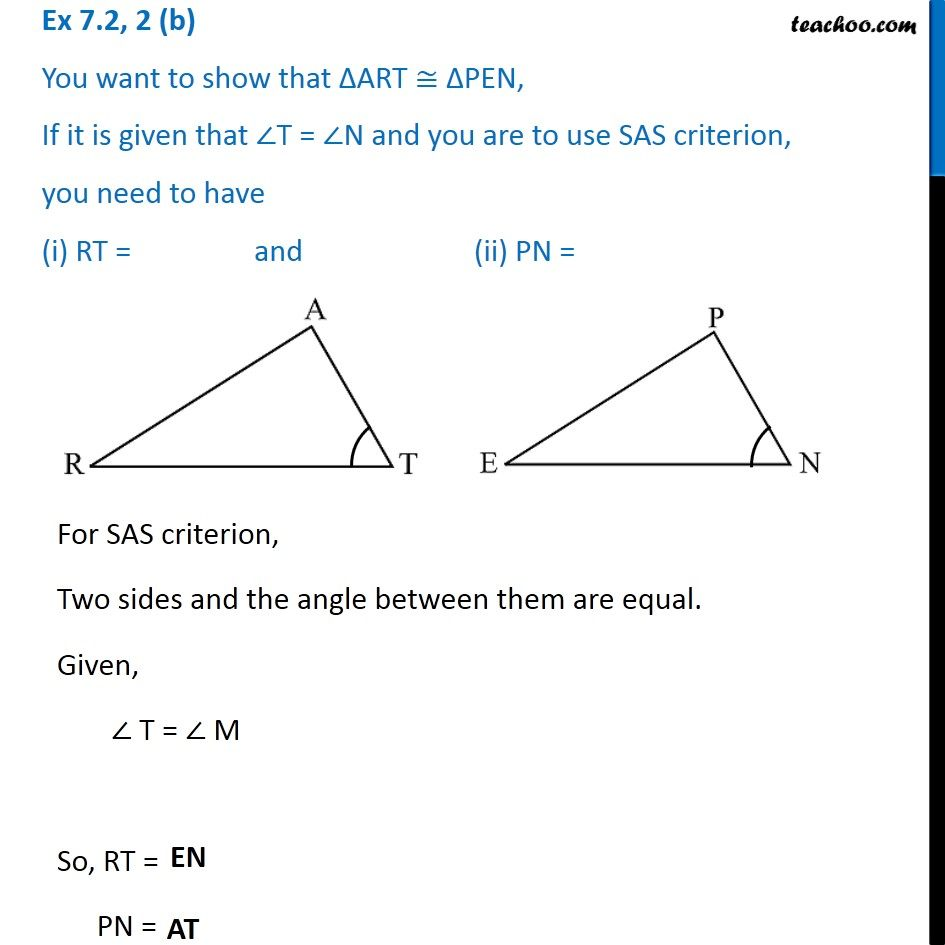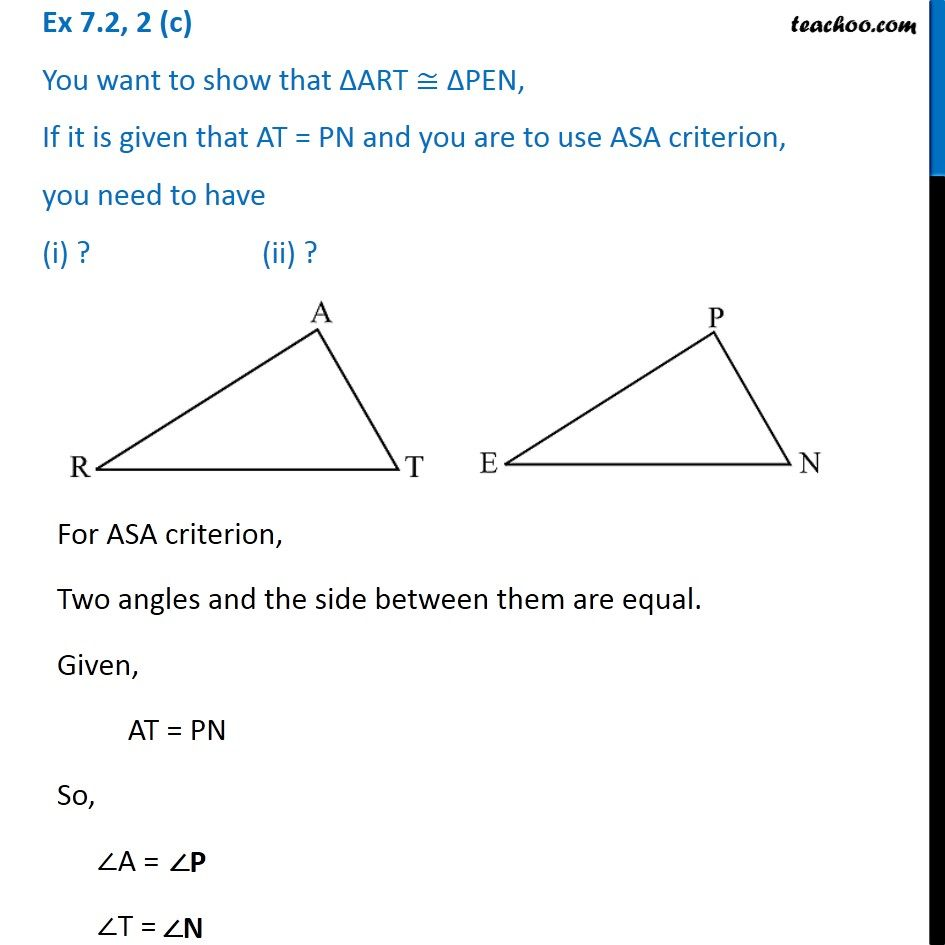Subscribe to our Youtube Channel - https://you.tube/teachoo

1. Chapter 7 Class 7 Congruence of Triangles
2. Serial order wise
3. Ex 7.2

Transcript

Ex 7.2, 2 (a) You want to show that ∆ART ≅ ∆PEN, If you have to use SSS criterion, then you need to show (i) AR = (ii) RT = (iii) AT = For SSS criteria, All three sides must be equal Therefore, AR = RT = AT = Ex 7.2, 2 (b) You want to show that ∆ART ≅ ∆PEN, If it is given that ∠T = ∠N and you are to use SAS criterion, you need to have (i) RT = and (ii) PN =For SAS criterion, Two sides and the angle between them are equal. Given, ∠ T = ∠ M So, RT = PN = Ex 7.2, 2 (c) You want to show that ∆ART ≅ ∆PEN, If it is given that AT = PN and you are to use ASA criterion, you need to have (i) ? (ii) ?For ASA criterion, Two angles and the side between them are equal. Given, AT = PN So, ∠A = ∠T =

Ex 7.2